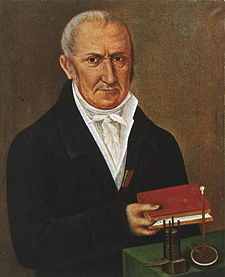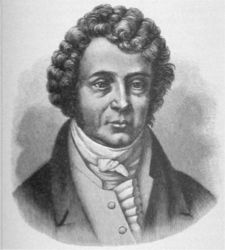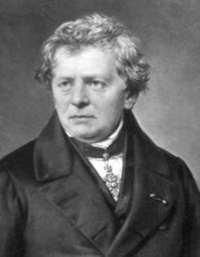#Drama! Intrigue! Geekiness!

## October 27, 2007

### High-School Physical

If you replace the pictures of these dudes with their names in the equation below, you will see a very fundamental physics equation. Identify the equation and name the three. ( if you get the equation, naming them is a piece of cake)=xCracked by: srikanth , piezocake , Poornima , vaibhav008 , sidsen , bs , sridhar , Atul Mathew , Ganesh Prasad , Gammafunction , Quehin , Shakeel , Goutham , rajeshvj , iamniks , nihit , Prasad and BiGFooT.

V=IR
1. Alessandro Volta
2. André-Marie Ampère
3. Georg Simon Ohm

### 18 Responses to “High-School Physical”

1. srikanth
 Volta, Ampere and Ohm V = IR Awesome question. Best I've seen on here so far :-)

2. piezocake
 V=IR. Volta, Ampere and Ohm.

3. Poornima
 V=IR 1. Alessandro Volta 2. André-Marie Ampère 3. Georg Simon Ohm

4. vaibhav008
 v = i r alessandro volta mr. ampere georg ohm

5. sidsenandre marie ampere in to georg ohm gets you a alesandro volta, is it just me or does that phrasing really sound a bit inappropriate for public forum discussions :)

6. bs
 hehe Alessandro Volta = Andreas Ampere * Georg Ohm V = I*R.

7. sridhar
 The equation is V=IR So the people are George ohm, Something Ampere and Alessandro Volta from right to left!!!! Finally, a question thats not just for tech quizzers!

8. Atul MathewV=IR Alessandro Volta=Ampere x Ohm

9. Ganesh Prasad
 V = IR Alessandro Volta(V) = Andre-Marie Ampere(I) X George Simon Ohm(R)

10. GammafunctionV=I.R Alessandro Volta =  Andre-Marie Ampere.Georg Ohm

11. Quehin
 V = IR Volta, Ampere, Ohm.

12. Shakeel
 The equation is V=I*R.  Persons from left are Alessandro Volta,Andre-Marie Ampere,and Georg Simon Ohm.

13. Goutham
 V=IR Volta, Ampere and Ohm

14. rajeshvjEquation: Ohm's Law i.e.  V = I x R 1. Alessandro Volta 2. Andre Marie Ampere 3. Georg Simon Ohm

15. iamniks
 V=IR V in Volt I in Ampere R in Ohm

16. nihitalessandro volta = andré-marie ampère x georg ohm

17. Prasad
 Nice one…atlast those 2 yrs of 'tronics pay off. Allesandro Volta = Ampere X George Ohm

18. BiGFooT
 Eqn : V =IR , Dudeshes(sic) are : Alessandro Volta, Ampere, Ohm

« Previous « Life, what is it but a dream? « | » Fundamental » Next »